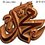# How do I start?

$$I\quad came\quad across\quad the\quad following\quad question:\\ Prove\quad that,\quad given\quad any\quad 52\quad integers,\quad there\quad exist\quad two\quad ofthem\quad whose\quad sum,\quad or\quad else,\quad whose\quad difference,\quad is\quad divisible\quad by\quad 100.$$

$I\quad just\quad started\quad using\quad latex.\quad Sorry$Note by Ceesay Muhammed
6 years, 6 months ago

This discussion board is a place to discuss our Daily Challenges and the math and science related to those challenges. Explanations are more than just a solution — they should explain the steps and thinking strategies that you used to obtain the solution. Comments should further the discussion of math and science.

When posting on Brilliant:

• Use the emojis to react to an explanation, whether you're congratulating a job well done , or just really confused .
• Ask specific questions about the challenge or the steps in somebody's explanation. Well-posed questions can add a lot to the discussion, but posting "I don't understand!" doesn't help anyone.
• Try to contribute something new to the discussion, whether it is an extension, generalization or other idea related to the challenge.

MarkdownAppears as
*italics* or _italics_ italics
**bold** or __bold__ bold
- bulleted- list
• bulleted
• list
1. numbered2. list
1. numbered
2. list
Note: you must add a full line of space before and after lists for them to show up correctly
paragraph 1paragraph 2

paragraph 1

paragraph 2

[example link](https://brilliant.org)example link
> This is a quote
This is a quote
    # I indented these lines
# 4 spaces, and now they show
# up as a code block.

print "hello world"
# I indented these lines
# 4 spaces, and now they show
# up as a code block.

print "hello world"
MathAppears as
Remember to wrap math in $$ ... $$ or $ ... $ to ensure proper formatting.
2 \times 3 $2 \times 3$
2^{34} $2^{34}$
a_{i-1} $a_{i-1}$
\frac{2}{3} $\frac{2}{3}$
\sqrt{2} $\sqrt{2}$
\sum_{i=1}^3 $\sum_{i=1}^3$
\sin \theta $\sin \theta$
\boxed{123} $\boxed{123}$

Sort by:

$Bad English In the question. Sorry$

- 6 years, 6 months ago

It's something about the pigeonhole principle. Sorry no time now, got to go to class.

- 6 years, 6 months ago

Since we need to check the divisibility by $100$, we'll only concentrate on the last two digits of the numbers.

The only possible last two digits are $\text{00, 01, 02, ..........., 98, and 99}$, a total of $100$ last two digits.

If the last two digits of any $2$ numbers are the same, their difference will be divisible by $100$.

Hence, we know that the last two digits of all numbers are different. Now, we divide the numbers into pairs that add up to $100$. The pairs are $(01,99)$, $(02,98)$, ........., $(49,51)$. And we can also use $0$ and $50$ only once.

Total number of pairs is $49$ and $2$ extra numbers. This makes a total of $51$ numbers, but we need $52$.

Hence, we must either use two numbers from any pair (making the sum divisible by $100$), or we need to use a number twice (making the diifference divisible by $100$).

$\Large \mathbb{QED}$

- 6 years, 6 months ago

@Ceesay Muhammed you don't need to latex the whole text; see the Beginner Latex Guide, it'll help a lot.

- 6 years, 6 months ago

Q.E.D. is an understatement :D

- 6 years, 6 months ago

LOL It's not even a statement... :-P

- 6 years, 6 months ago